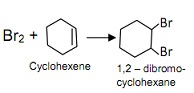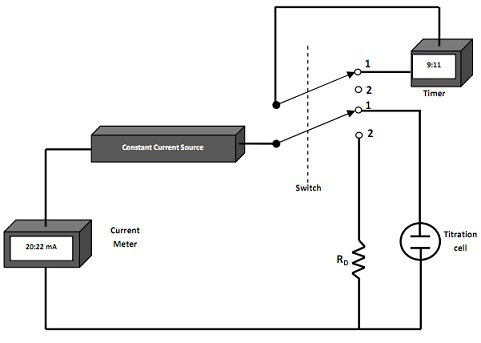#### Coulometry, Chemistry tutorial

Introduction

Coulometry is an electro-analytical method of analysis. Faraday's laws of electrolysis form the basis of quantitative coulometric analysis. You should recall that according to this law: The weight of substance liberated during electrolysis is directly proportional to the quantity of electricity passed. Also the weight of substances liberated by the same quantity of electricity is in direct proportion to their equivalent weights. Coulometric methods are performed by measuring the quantity of electrical charge required to convert a sample of analyte quantitatively to a different oxidation state.

Definition

Coulometry is an electrolytic method of analysis that is based on measuring the number of electrons used in a chemical reaction.

A Typical Example of Coulometric Process

In coulometry, cyclohexene might be titrated by Br2 that is produced through electrolytic oxidation of Bromide (Br-). For example 2 Br- → Br2 + 2e-

Then;In the above reactions, sufficient of bromine is produced to react through all of the cyclohexene. Therefore, the number of moles of electrons released is twice that of Br2 and therefore, twice the moles of cyclohexene.

In coulometry, the reaction between Br2 and cyclohexene is carried out using a coulometric apparatus. During the procedure, enough Br2 is produced through the platinum electrode positioned at the left side. The generated Br2 then reacts by cyclohexene. If all of the cyclohexene has been reacted, the concentration of Br2 in the solution abruptly rises that specifies the end of the reaction.

The increase in concentration of Br2 is detected through measuring the electric current that is applied in the procedure. Therefore, a small voltage is applied between the 2 electrodes but this isn't sufficient to electrolyze the solute. Whenever the concentration of Br2 abruptly raises current flows as a consequence of the reaction taking place in together cathode and anode. Therefore, the rapid enhance in current is taken as the end point of cyclohexene titration.

Types of coulometry

There are 2 general kinds of coulometric techniques namely: Controlled potential and constant current.

Constant current coulometry

In this kind of analysis, a steady current is applied whenever the power is attached to the electrodes. This technique is termed coulometric titration. If the current is recognized, the time needed for entire reaction is calculated in order to count the coulombs.

For example q = It

The power provides is built to automatically calculate the time of operation. When the current is multiplied through the time taken, the number of coulombs employed in the titration is attained.

Controlled potential coulometry (potentiostatic)

In this technique, the potential of the working electrode is kept at steady level in these a way that only the analyte is dependable for conducting charge across the electrode and solution interface. The charge needed to change the analyte into product is computed through recording and integrating the current against time curve during the electrolytic process. For example Z

For example q = 0t Idt

In this case the current is initially high and decreases exponentially as the concentration of the analyte sample decreases. Thus, the current decreases with time. Also the power supply contains a circuit that automatically integrates equation (1) to calculate the number of coulombs applied. Controlled potential coulometry is more selective than the constant current. As the current decays or decreases exponentially, the equivalence point is never reached. But the equivalence point can be obtained by setting the current decay to an arbitrary value. Example, the current will be 1% of its initial value when 99% of the analyte sample is consumed. On the other hand, the current will be 0.1% when 99.9% of the analyte has been used up.

A Typical example of Coulometric Calculations

Assuming that a 2000 cm3 of solution containing 0.7113 mg of cyclohexene/cm3 is to be titrated against bromine. If the coulometer is activated at a steady current of 5.820mA, what would be the time needed for entire titration?

(Molar mass of cyclohexene = 82.146; 1F = 96485C)

In this case, the quantity of cyclohexene is calculated as follows:

(2000ml*0.7113 mg/ml)/82.146 mg/mmol = 17.318 mol ≡ 0.0173 m mol

Since 82.146 is the molecular weight of cyclohexene and each mole of cyclohexene requires one mole of Br2, which in turn requires 2 moles of electrons to flow through the circuit.

∴ for 0.01732 mmol of hexane to react, 0.03463 mmol of electrons must flow.

Moles of e- = lt / F

t = Moles of e- * F/ 1

t= (0.03463 * 96485) c/mol/ 5.820*10-3 c/s = 574.102 s/mol

Current Efficiency requirements

In all coulometric methods 100% current efficiency is needed. That is each Faraday of electricity must bring about a chemical transform in the analyte equivalent to 1 mole of electron. As we know that 100% current efficiency can be attained with no direct participation of the analyte in electron transport at an electrode. For instance, Chloride ion (Cl-) might be simply computed using controlled potential coulometry or coulometric titrations through silver ion (Ag+) at a silver anode. The silver ion then reacts through chloride ion to form a precipitate or deposit of silver chloride. The quantity of electricity needed to complete the silver chloride formation provides as analytical variable.

In this case, 100% current competence is realized since the number of moles of electrons is basically equivalent to the number of moles of chloride ion in the example, even though such ions don't react straight at the electrode surface.

Instrumentation

The equipment needed for a coulometric titration consists of a source of steady current, a titration cell, a switch, a timer and a machine for monitoring current. When the switch is shifted to position 1, the timer starts and begins a current in the titration cell. When the switch is shifted to position 2, the electrolysis and the timer are stopped. Through the switch in this position, the current maintains to be drawn from the source and passes through a dummy resistor RD that has about the same electrical resistance as the cell. This arrangement guarantees continuous operation of the source that aids in maintaining a stable current.Fig: Conceptual diagram of a coulometric titration apparatus.

But the instrument utilized for controlled potential coulometry consists of an electrolytic cell, a potentiostat and a machine for determining the transform devoured through the analyte example. 2 kinds of cells are employed. The 1st consists of a platinum gauze working electrode, a platinum wire counter electrode and a saturated calomel reference electrode. The counter electrode is separated from the analyte solution through a salt bridge that encloses the similar electrolyte as the solution being analyzed. This bridge is required to prevent the reaction products shaped at the counter electrodes from diffusing into the analyte solution and interfering.

The 2nd cell is made of mercury cathode. This is helpful for separating simply diminished elements as a preliminary step in analysis. This electrode is as well helpful in the coulometric determination of numerous metallic cations that form metals that are soluble in mercury. In this application, there is only little evolution of H2 gas even at high applied potentials.

Advantages of Coulometric Titration over the Conventional Titrations

Coulometric titration has a batch of benefits over a normal volumetric titration. Amongst the benefits is the abolition of the problems connected through the preparation, standardization and storage of standard solutions. This benefit is particularly significant via reagents that aren't stable these as chlorine, bromine, and so on. Another benefit is that coulometric technique excels when tiny amounts of analyte is to be employed in the titration. But through conventional titration, it is inaccurate and inconvenient to employ extremely dilute solutions and small volumes.

In coulometric procedure, a single constant current source provides reagents for precipitation, complex formation, and neutralization or redox titrations. But this isn't possible in normal volumetric titration. At last coulometric titrations are more adaptable and more precise than the conventional volumetric procedure.

Application

Coulometric techniques have been utilized to find out numerous elements in inorganic compounds and synthesize organic compounds. But usually, coulometric titration is applied in:

• Neutralization titration
• Precipitation and complex formation reactions for instance coulometric titration with EDTA are carried out by reduction of the ammine mercury (II) EDTA chelate at mercury cathode.
• Oxidation - reduction (redox) titration - coulometric titrations have been applied for many redox titrations

Tutorsglobe: A way to secure high grade in your curriculum (Online Tutoring)